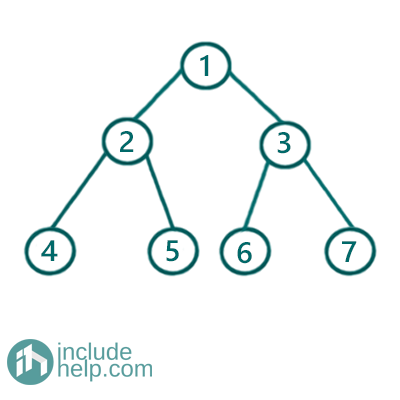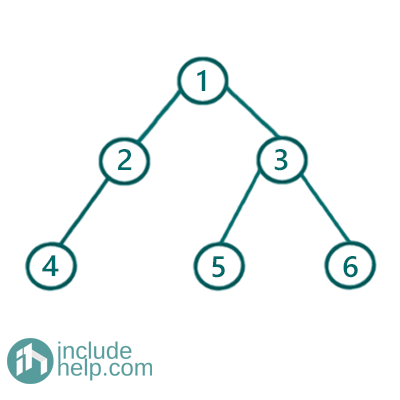# Check if the given tree is perfect binary tree or not

In this article we are going to see whether the given tree is perfect binary tree or not.
Submitted by Radib Kar, on August 08, 2020

In the previous tutorial on Perfect Binary Tree, we saw that the Perfect Binary Tree is a special kind of binary tree where each and every internal node has exactly 2 children and all the leaf nodes lie in the same level.

For example, the below binary tree is a perfect binary tree whereas the second one is not.

Example 1:Figure 1: Perfect Binary Tree

The above example is a Perfect Binary Tree as every internal node in this have exact two children and all the leaf nodes lie in same level.

Example 2:Figure 2: Binary Tree which is Not a Perfect Binary Tree

The above binary tree is not a perfect binary tree as all its internal nodes don't have exactly 2 children though all leaf nodes lie in same level. The node having value 4 has only one child.

To check whether a binary tree is perfect or not can be done by using perfect tree properties. We saw that if number of total nodes in the tree is N & height of the tree is h then, for a perfect binary Tree: N = 1+2+22+…+2h =2h + 1 – 1. So, if we know the height of the binary tree and number of total nodes we can apply the above formula and check whether the tree is perfect or not. . So we simply count the total number of nodes & height of the tree using recursion and check whether it follows the rule.

C++ Implementation:

```#include <bits/stdc++.h>
using namespace std;

class TreeNode {
public:
int val;
TreeNode* left;
TreeNode* right;

TreeNode(int v)
{
val = v;
left = NULL;
right = NULL;
}
};

int height(TreeNode* root)
{
if (!root)
return 0;

return 1 + max(height(root->left), height(root->right));
}

int count_no_of_nodes(TreeNode* root)
{
if (!root)
return 0;

return 1 + count_no_of_nodes(root->left) + count_no_of_nodes(root->right);
}

//Perfect Binary Tree checking
bool is_Perfect_Binary_Tree(TreeNode* root)
{
int h = height(root) - 1;
int total_no_of_nodes = count_no_of_nodes(root);
return pow(2, h + 1) - 1 == total_no_of_nodes;
}

int main()
{
//Example 1 Tree is built
TreeNode* root1 = new TreeNode(1);

root1->left = new TreeNode(2);
root1->right = new TreeNode(3);
root1->left->left = new TreeNode(4);
root1->left->right = new TreeNode(5);
root1->right->left = new TreeNode(6);
root1->right->right = new TreeNode(7);

if (is_Perfect_Binary_Tree(root1))
cout << "The tree is Example 1 is a Perfect Binary Tree\n";
else
cout << "The tree is Example 1 is not a Prefect Binary Tree\n";

//Example 2 Tree is built
TreeNode* root2 = new TreeNode(1);

root2->left = new TreeNode(2);
root2->right = new TreeNode(3);
root2->left->left = new TreeNode(4);
root2->right->left = new TreeNode(5);
root2->right->right = new TreeNode(6);

if (is_Perfect_Binary_Tree(root2))
cout << "The tree in Example 2 is a Perfect Binary Tree\n";
else
cout << "The tree in Example 2 is not a Perfect Binary Tree\n";

return 0;
}
```

Output:

```The tree is Example 1 is a Perfect Binary Tree
The tree in Example 2 is not a Perfect Binary Tree
```